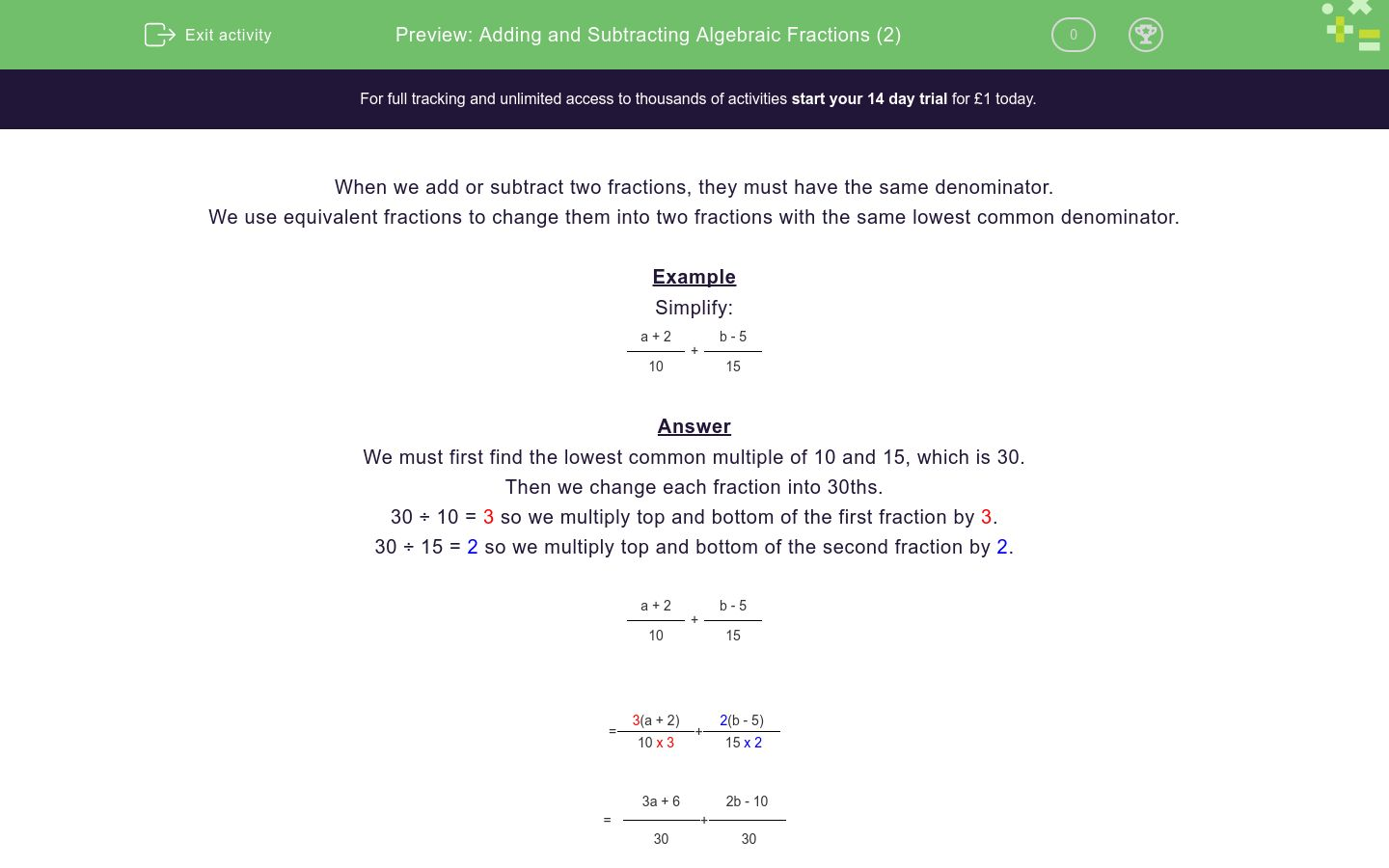# Adding and Subtracting Algebraic Fractions (2)

In this worksheet, students add or subtract algebraic fractions giving their answer as a single fraction.Key stage:  KS 4

Curriculum topic:  Algebra

Curriculum subtopic:  Simplify/Manipulate Algebraic Expressions/Fractions

Difficulty level:### QUESTION 1 of 10

When we add or subtract two fractions, they must have the same denominator.

We use equivalent fractions to change them into two fractions with the same lowest common denominator.

Example

Simplify:

 a + 2 + b - 5 10 15

We must first find the lowest common multiple of 10 and 15, which is 30.

Then we change each fraction into 30ths.

30 ÷ 10 = 3 so we multiply top and bottom of the first fraction by 3.

30 ÷ 15 = 2 so we multiply top and bottom of the second fraction by 2.

 a + 2 + b - 5 10 15

 = 3(a + 2) + 2(b - 5) 10 x 3 15 x 2

 = 3a + 6 + 2b - 10 30 30

 = 3a + 2b + 6 - 10 30

 = 3a + 2b - 4 30

Simplify the following and select the correct answer:

 a + 2 + a + 5 10 15

 1 5a + 7 2. 5a + 16 3. 2a + 7 15 30 25

1.

2.

3.

Simplify the following and select the correct answer:

 a + 2 + a - 2 2 3

 1 5a + 2 2. 2a 3. 5a + 2 5 5 6

1.

2.

3.

Simplify the following and select the correct answer:

 a + 3 + a - 5 2 3

 1 5a + 2 2. 2a - 2 3. 5a - 1 6 5 6

1.

2.

3.

Simplify the following and select the correct answer:

 a + 3 + a + 4 4 6

 1 5a + 17 2. 5a - 2 3. 5a + 7 12 5 12

1.

2.

3.

Simplify the following and select the correct answer:

 a + 4 + a + 3 4 6

 1 5a + 17 2. 5a - 2 3. 5a + 18 12 5 12

1.

2.

3.

Simplify the following and select the correct answer:

 a + 4 + a - 3 4 5

 1 9a + 5 2. 9a + 8 3. 2a + 18 20 20 9

1.

2.

3.

Simplify the following and select the correct answer:

 a + 4 + a - 3 4 8

 1 3a + 5 2. 9a + 8 3. 2a + 18 8 32 12

1.

2.

3.

Simplify the following and select the correct answer:

 a - 4 + a - 2 4 2

 1 2a - 6 2. 9a + 8 3. 3a - 8 6 8 4

1.

2.

3.

Simplify the following and select the correct answer:

 a - 4 + a - 2 4 12

 1 2a - 6 2. 2a - 7 3. a - 3 16 6 8

1.

2.

3.

Simplify the following and select the correct answer:

 a + 2 + a - 3 6 9

 1 2a - 1 2. 5a 3. 5a - 1 15 18 18

1.

2.

3.

• Question 1

Simplify the following and select the correct answer:

 a + 2 + a + 5 10 15

 1 5a + 7 2. 5a + 16 3. 2a + 7 15 30 25

2.
• Question 2

Simplify the following and select the correct answer:

 a + 2 + a - 2 2 3

 1 5a + 2 2. 2a 3. 5a + 2 5 5 6

3.
• Question 3

Simplify the following and select the correct answer:

 a + 3 + a - 5 2 3

 1 5a + 2 2. 2a - 2 3. 5a - 1 6 5 6

3.
• Question 4

Simplify the following and select the correct answer:

 a + 3 + a + 4 4 6

 1 5a + 17 2. 5a - 2 3. 5a + 7 12 5 12

1.
• Question 5

Simplify the following and select the correct answer:

 a + 4 + a + 3 4 6

 1 5a + 17 2. 5a - 2 3. 5a + 18 12 5 12

3.
• Question 6

Simplify the following and select the correct answer:

 a + 4 + a - 3 4 5

 1 9a + 5 2. 9a + 8 3. 2a + 18 20 20 9

2.
• Question 7

Simplify the following and select the correct answer:

 a + 4 + a - 3 4 8

 1 3a + 5 2. 9a + 8 3. 2a + 18 8 32 12

1.
• Question 8

Simplify the following and select the correct answer:

 a - 4 + a - 2 4 2

 1 2a - 6 2. 9a + 8 3. 3a - 8 6 8 4

3.
• Question 9

Simplify the following and select the correct answer:

 a - 4 + a - 2 4 12

 1 2a - 6 2. 2a - 7 3. a - 3 16 6 8

2.
• Question 10

Simplify the following and select the correct answer:

 a + 2 + a - 3 6 9

 1 2a - 1 2. 5a 3. 5a - 1 15 18 18